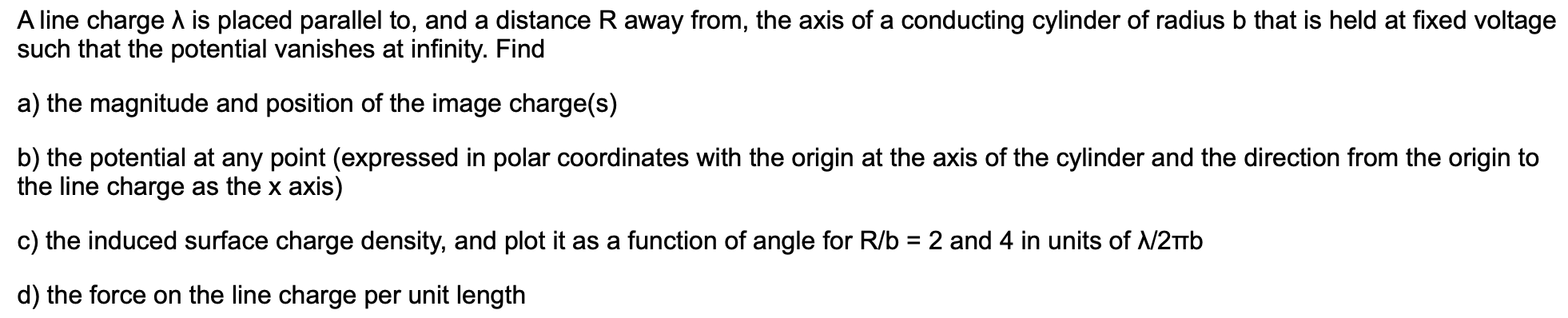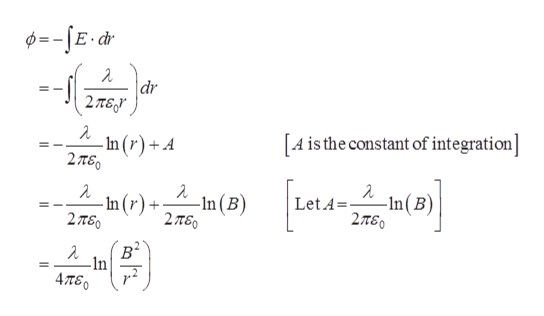# A line charge is placed parallel to, and a distance R away from, the axis of a conducting cylinder of radius b that is held at fixed voltagesuch that the potential vanishes at infinity. Finda) the magnitude and position of the image charge(s)b) the potential at any point (expressed in polar coordinates with the origin at the axis of the cylinder and the direction from the origin tothe line charge as the x axis)c) the induced surface charge density, and plot it as a function of angle for R/b2 and 4 in units of N2TTBd) the force on the line charge per unit length

Question

It's an electromagnetic problem. 3help_outlineImage TranscriptioncloseA line charge is placed parallel to, and a distance R away from, the axis of a conducting cylinder of radius b that is held at fixed voltage such that the potential vanishes at infinity. Find a) the magnitude and position of the image charge(s) b) the potential at any point (expressed in polar coordinates with the origin at the axis of the cylinder and the direction from the origin to the line charge as the x axis) c) the induced surface charge density, and plot it as a function of angle for R/b 2 and 4 in units of N2TTB d) the force on the line charge per unit length fullscreen
check_circleExpert Solution
Step 1

a)

Refer the diagram below.

Step 2

Using Gauss law,

Step 3

Potential ...help_outlineImage Transcriptionclosedr dr 2T6 A is the constant of integration] -In (r) +A 2TS0 -In(B) 2πε LetA -In (B) -In (r) + 2πο 2π6ο B В* In 4πεο II fullscreen

### Want to see the full answer?

See Solution

#### Want to see this answer and more?

Solutions are written by subject experts who are available 24/7. Questions are typically answered within 1 hour*

See Solution
*Response times may vary by subject and question
Tagged in

### Science